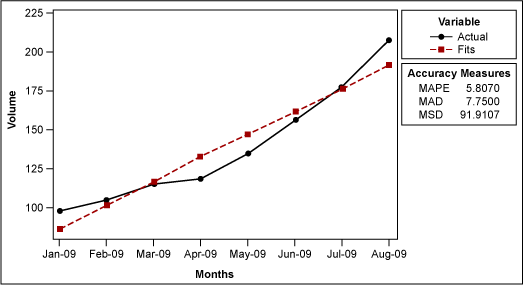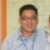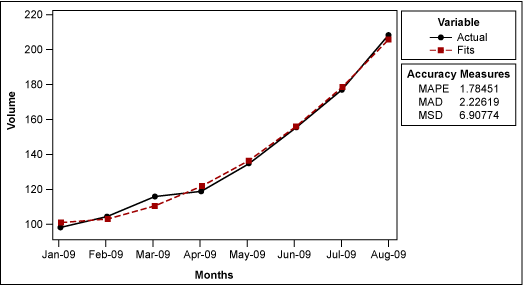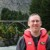# Making Sense of Time Series ForecastingByChew Jian Chieh

It is a common scenario: A practitioner has sales data for the past several months and wants to forecast next month’s sales volume. This type of forecasting can help manufacturers and distributors ensure they have enough product to meet customer demands. But how is this forecasting done? Statistical analysis software offers two ways to plot the data in order to make a forecast: 1) a linear trend model or 2) a quadratic trend model. It is important for practitioners to understand both methods, as each can be beneficial, depending on the type of process being analyzed.

### Part 1 – Linear Trend

The following explanations use the sample data shown in Table 1.

Table 1: Sample Volume Data

 Month Volume Jan. 2009 98 Feb. 2009 105 March 2009 116 April 2009 119 May 2009 135 June 2009 156 July 2009 177 Aug. 2009 208

To begin, use statistical analysis software to create a time series plot with a linear trend analysis (Figure 1).Figure 1: Trend Analysis Plot for Volume – Linear Trend Model

The software will generate a fitted line using the equation Yt = 71.43 + (15.1 x t). The t represents the time period during which each data point was collected – i.e., the first time period is 1, the second is 2 and so on. Hence, if someone wants to know the fitted value for January 2009, it is 71.43 +15.1*(1) = 86.53.

Table 2: Fitted Values for Past Months

Handpicked Content:   Thinking Two Moves Ahead with Analytical Tools
 Month Volume Fitted Value t Jan. 2009 98 86.53 1 Feb. 2009 105 101.63 2 March 2009 116 116.73 3 April 2009 119 131.83 4 May 2009 135 146.93 5 June 2009 156 162.03 6 July 2009 177 177.13 7 Aug. 2009 208 192.23 8

To forecast for September 2009, the practitioner would get 207.33 (71.43 + (15.1 x 9)).

But how does the software get the equation Yt = 71.43 + (15.1 x t)? It is nothing but linear regression. If practitioners used the linear regression function in their statistical analysis software instead, using volume for Y and the t (1, 2, 3, 4, etc.) for X they would get the same equation:

Regression Analysis: Volume versus t

The regression equation is
Volume = 71.4 + 15.1 t

Predictor Coef SE Coef T P
Constant 71.429 8.626 8.28 0.000
t 15.071 1.708 8.82 0.000

S = 11.0701 R-Sq = 92.8% R-Sq(adj) = 91.7%

Analysis of Variance

Source DF SS MS F P
Regression 1 9540.2 9540.2 77.85 0.000
Residual Error 6 735.3 122.5
Total 7 10275.5

Another potentially confusing element of the linear trend plot is the forecast accuracy measures: MAD, MAPE and MSD. These are used to determine how well the trend will accurately predict the future volume.

MAD stands for mean absolute deviation, which is the average of the absolute deviations. An absolute deviation is the absolute value of the actual data minus the fitted value (Table 3).

Handpicked Content:   Experiential Learning of Lean Six Sigma with Marbles and Toy Cars

Table 3: Sample Data Including Absolute Deviation

 Month Volume Fitted Value t Absolute Deviation Jan. 2009 98 86.53 1 11.47 Feb. 2009 105 101.63 2 3.37 March 2009 116 116.73 3 0.73 April 2009 119 131.83 4 12.83 May 2009 135 146.93 5 11.93 June 2009 156 162.03 6 6.03 July 2009 177 177.13 7 0.13 Aug. 2009 208 192.23 8 15.77 Sum 62.26 n 8 MAD 7.7825

The best fitted line should have zero MAD; the larger the MAD, the worse the model. For instance, instead of using linear regression to generate a forecast, a practitioner might base the forecast on last month’s volume. This generates a different MAD value (Table 4).

Table 4: Forecast Using Last Month’s Volume

 Month Volume Fitted Value t Absolute Deviation Jan. 2009 98 80 1 18 Feb. 2009 105 98 2 7 March 2009 116 105 3 11 April 2009 119 116 4 3 May 2009 135 119 5 16 June 2009 156 135 6 21 July 2009 177 156 7 21 Aug. 2009 208 177 8 31 Sum 128 n 8 MAD 16

The MAD value allows the practitioner to conclude that the model generated by linear regression is better than the model generated by last month’s volume.

MSD

The linear trend plot also uses the accuracy measure MSD, which stands for mean square deviation. It is very similar to MAD, but instead of summing the absolute deviations, this measure sums up the squared deviations (Table 5).

Table 5: Sample Data Including Squared Deviations

 Month Volume Fitted Value t Absolute Deviation Squared Deviations Jan. 2009 98 86.5 1 11.47 132.25 Feb. 2009 105 101.571429 2 3.37 11.75510204 March 2009 116 116.642857 3 0.73 0.413265306 April 2009 119 131.714286 4 12.83 161.6530612 May 2009 135 146.785714 5 11.93 138.9030612 June 2009 156 161.857143 6 6.03 34.30612245 July 2009 177 176.928571 7 0.13 0.005102041 Aug. 2009 208 192 8 15.77 256 Sum 735.2857143 n 8 MSD 91.91071429

So what is the difference? MSD weights large deviations more heavily because it takes the square of the deviations. In general, MSD is preferred over MAD because there seems to be more theoretical support for it.

MAPE

The third accuracy measure is MAPE, or mean absolute percentage error. It is calculated by taking the absolute deviation and dividing it by the data (the volume in this case) to get the percent error (Table 6).

Handpicked Content:   Interpreting Anomalies Correctly Can Help Avoid Waste

Table 6: Sample Data Including Absolute Percent Error

 Month Volume Fitted Value t Absolute Deviation Absolute Percent Error Jan. 2009 98 86.5 1 11.5 11.73469388 Feb. 2009 105 101.571429 2 3.428571429 3.265306122 March 2009 116 116.642857 3 0.642857143 0.554187192 April 2009 119 131.714286 4 12.71428571 10.68427371 May 2009 135 146.785714 5 11.78571429 8.73015873 June 2009 156 161.857143 6 5.8571428571 3.754578755 July 2009 177 176.928571 7 0.071428571 0.040355125 Aug. 2009 208 192 8 16 7.692307692 Sum 46.4558612 n 8 MAPE 5.80698265

MAPE is typically used less often than MAD and MSE.

### Part 2 – Quadratic Trend

If the practitioner suspects the trend in volume is quadratic rather than linear (meaning the volume is increasing at a faster rate than it would with linear proportion), they would create a plot with a quadratic trend in their statistical analysis software (Figure 2).Figure 2: Trend Analysis Plot for Volume – Quadratic Trend Model

It is no surprise that this model is better than the linear model from a MAPE, MAD and MSD perspective because it is a more complex model, requiring more terms. However, it is generally impossible to say which is the correct model to use. The decision requires a judgment call based on the practitioner’s understanding of the process.

The equation used with the quadratic trend is Yt = 101.61 – (3.04 x t) + (2.012 x t2), Once again, this equation is reached through regression analysis. To complete this regression using statistical analysis software, the practitioner first needs to square the t series (Table 7).

Table 7: Sample Data with T Value Squared

 Month Volume t t2 Jan. 2009 98 1 1 Feb. 2009 105 2 4 March 2009 116 3 9 April 2009 119 4 16 May 2009 135 5 25 June 2009 156 6 36 July 2009 177 7 49 Aug. 2009 208 8 64

Next, the practitioner performs a multiple regression of the volume on t and t2. The result is:

Regression Analysis: Volume versus t, t sqr

The regression equation is
Volume = 102 – 3.04 t + 2.01 t sqr

Predictor Coef SE Coef T P
Constant 101.607 4.638 21.91 0.000
t -3.036 2.365 -1.28 0.256
t sqr 2.0119 0.2565 7.84 0.001

S = 3.32451 R-Sq = 99.5% R-Sq(adj) = 99.2%

Analysis of Variance

Source DF SS MS F P
Regression 2 10220.2 5110.1 462.35 0.000
Residual Error 5 55.3 11.1
Total 7 10275.5

Source DF Seq SS
t 1 9540.2
t sqr 1 680.0

The equation is the same as the one generated when creating the quadratic trend analysis plot.

##### Chew Jian Chieh

1.peiling

how to get 71.43 and 15.1 ?

2.Carl Berardinelli

Nice summary! Thanks for sharing your insights.

3.4.Hemant

Excellent and easy explanation.